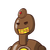# 8x-5 Let f (x) = CX + 6 6 for x–,C+0. с (a) The line x = 3 is a vertical asymptote to t

8x-5
Let f (x) =
CX + 6
6
for x–,C+0.
с
(a)
The line x = 3 is a vertical asymptote to the graph of f. Find the value of c.

(b)
Write down the equation of the horizontal asymptote to the graph of f.

(c)
The line y=k, where ke R intersects the graph of f(x) at exactly one point. Find
the possible values of k.


### 1 thought on “8x-5<br /> Let f (x) =<br /> CX + 6<br /> 6<br /> for x–,C+0.<br /> с<br /> (a)<br /> The line x = 3 is a vertical asymptote to t”

1.